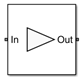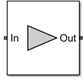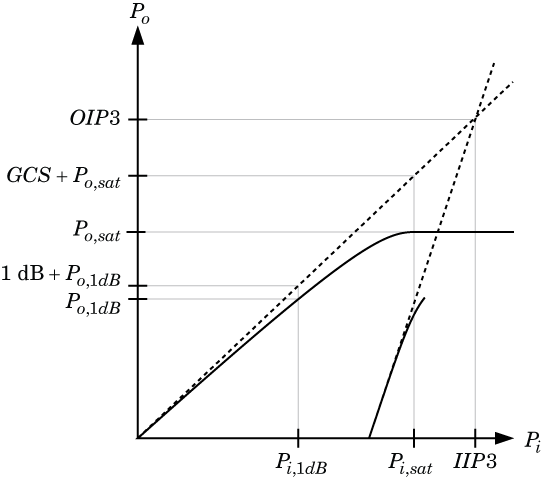Amplifier

Model amplifier in RF systems

• Library:
• RF Blockset / Circuit Envelope / Elements

•Description

Use the Amplifier block to model a linear or nonlinear amplifier, with or without noise. Defining the amplifier gain using a data source also defines input data visualization and modeling. Use the Main tab parameters to specify amplifier gain and noise using data sheet values, standard s2p files, S-parameters, or circuit envelope polynomial coefficients.

The amplifier is implemented as a polynomial, voltage-controlled voltage source (VCVS) except when the amplifier gain is obtained from a Data source. The VCVS includes nonlinearities that are described using parameters listed in the Nonlinearity tab. To model linear amplification, the amplifier implements the relation Vout = a1*Vin between the input and output voltages. The input voltage is Vi(t) = Ai(t)ejωt, and the output voltage is Vo(t) = Ao(t)ejωt at each carrier w = 2πf in the RF Blockset™ environment.

In case the amplifier gain is obtained from a data source, amplifier implementation is based on S-parameter data.

Nonlinear amplification is modeled as a polynomial (with the saturation power computed automatically). It also produces additional intermodulation frequencies.

Amplifier block mask icons are dynamic and show the current state of the applied noise parameter. This table shows you how the icons on this block vary based on the state of the Noise figure (dB) parameter on the block.

Noise Figure (dB): 10Noise Figure (dB): 0Parameters

expand all

Main

Source parameter of the amplifier gain, specified as one of the following:

• Available power gainAvailable power gain parameter is used to calculate the linear voltage gain term of the polynomial VCVS, a1. This calculation assumes a matched load termination for the amplifier.

• Open circuit voltage gainOpen circuit voltage gain parameter is used as the linear voltage gain term of the polynomial VCVS, a1.

• Data source — When using the data source option, S11 and S22, are used as the input and output impedances. The data sources are specified using either Data file or Network-parameters or Rational model, depending on the value of Data source.

• Polynomial coefficients — The block implements a nonlinear voltage gain according to the specified polynomial coefficients

Available power gain of amplifier, specified as a scalar in dB. Specify the units from the corresponding drop-down list.

Dependencies

To enable this parameter, choose Available power gain in the Source of amplifier gain tab.

Open circuit voltage of amplifier, specified as a scalar in dB. Specify the units from the corresponding drop-down list.

Dependencies

To enable this parameter, choose Open circuit voltage gain in the Source of amplifier gain tab.

Data source, specified as one of the following:

• Data file — Name of a Touchstone file with the extension.s2p.

• Network-parameters — Provide Network parameter data such as S-parameters, Y-parameters, and Z-parameters with corresponding Frequency and Reference impedance (ohms) for the amplifier.

• Rational model — Provide values for Residues, Poles, and Direct feedthrough parameters which correspond to the equation for a rational model

$F\left(s\right)=\left(\sum _{k=1}^{n}\frac{{C}_{k}}{s-{A}_{k}}+D\right)\begin{array}{cc},& s=j2\pi f\end{array}$

In this rational model equation, each Ck is the residue of the pole Ak. If Ck is complex, a corresponding complex conjugate pole and residue must also be enumerated. This object has the properties C, A, and D. You can use these properties to specify the Residues, Poles, and Direct feedthrough parameters.

When the amplifier is nonlinear, the nonlinearity applies only to the S21 term of the scattering parameters representing the 2-port element. In this case, S21 is frequency-independent with its constant value being either the maximal value of S21, or the S21 value at an Operation frequency specified by the user. The other scattering parameters, S11, S12, and S22 remain the same as in the linear case.

Dependencies

To enable this parameter, select Data source in Source of amplifier gain tab.

Order of polynomial, specified as a vector.

The order of the polynomial must be less than or equal to 9. The coefficients are ordered in ascending powers. If a vector has 10 coefficients, [a0,a1,a2, ... a9], the polynomial it represents is:

Vout = a0 + a1Vin + a2Vin2 + ...  + a9Vin9

where a1 represents the linear gain term, and higher-order terms are modeled according to .

For example, the vector [a0,a1,a2,a3] specifies the relation Vout = a0 + a1V1 + a2V12 + a3V13. Trailing zeroes are omitted. So, [a0,a1,a2] defines the same polynomial as [a0,a1,a2, 0]. The default value of this parameter is [0,1], corresponding to the linear relation Vout = Vin.

Dependencies

To enable this parameter, select Polynomial coefficients in Source of amplifier gain tab.

Network parameter type, specified as S-parameters, Y-parameters, or Z-parameters.

Dependencies

To enable this parameter, first select Data source in Source of amplifier gain tab. Then, select Network-parameters in the Data source tab.

Input impedance of amplifier, specified as a scalar.

Dependencies

To enable this parameter, select Available power gain, Open circuit voltage gain, or Polynomial coefficients in Source of amplifier gain tab.

Output impedance of amplifier, specified as a scalar.

Dependencies

To enable this parameter, select Available power gain, Open circuit voltage gain, or Polynomial coefficients in Source of amplifier gain tab.

Name of network parameter data file, specified as a character vector.

Dependencies

To enable this parameter, first select Data source in Source of amplifier gain tab. Then, select Data file in Data source.

Frequency of network parameters, specified as a scalar in Hz.

Dependencies

To enable this parameter, first select Data source in Source of amplifier gain tab. Then, select Network-parameters in Data source.

Reference impedance of network parameters, specified as a scalar.

Dependencies

To enable this parameter, first select Data source in Source of amplifier gain tab. Then, select Network-parameters in Data source.

Residues in order of rational model, specified as a vector.

Dependencies

To enable this parameter, first select Data source in Source of amplifier gain tab. Then, select Rational model in Data source.

Poles in order of rational model, specified as a vector.

Dependencies

To enable this parameter, first select Data source in Source of amplifier gain tab. Then, select Rational model in Data source.

Direct feedthrough, specified as an array vector.

Dependencies

To enable this parameter, first select Data source in Source of amplifier gain tab. Then, select Rational model in Data source.

Select this option to specify operation frequency.

By default, this option is not selected.

Dependencies

To enable this parameter, first you should specify nonlinear Polynomial coefficients in Source of amplifier gain. Then select Piece-wise linear orColored in Noise distribution in the Noise pane.

Operation frequency, specified as a scalar or vector in Hz.

Dependencies

To enable this parameter, first you should select Specify operation frequency.

Select this option to ground and hide the negative terminals. Clear this parameter to expose the negative terminals. By exposing these terminals, you can connect them to other parts of your model.

By default, this option is selected.

Nonlinearity

Type of nonlinearity, specified as Even and odd order or Odd order.

• When you select Even and odd order, the amplifier can produce second- and third-order intermodulation frequencies in addition to a linear term.

• When you select Odd order, the amplifier generates only odd order intermodulation frequencies.

The linear gain determines the linear a1 term. The block calculates the remaining terms from the specified parameters. These parameters are IP3, 1-dB gain compression power, Output saturation power, and Gain compression at saturation. The number of constraints you specify determines the order of the model. The figure shows the graphical definition of the nonlinear amplifier parameters.Intercept points convention, specified a Input-referred, or Output-referred convention. Use this specification for the intercept points, 1-dB gain compression power, and saturation power.

Second-order intercept point, specified as a scalar.

Dependencies

To set this parameter, select Even and odd order in Nonlinear polynomial type.

Third-order intercept point, specified as a scalar.

1-dB gain compression power, specified as a scalar.

Dependencies

To set this parameter, select Odd order in Nonlinear polynomial type.

Output saturation power, specified as scalar. The block uses this value to calculate the voltage saturation point used in the nonlinear model. In this case, the first derivative of the polynomial is zero, and the second derivative is negative.

Dependencies

To set this parameter, select Odd order in Nonlinear polynomial type.

Gain compression at saturation, specified as scalar.

When Nonlinear polynomial type is Odd order, specify the gain compression at saturation.

Dependencies

To set this parameter, first select Odd order in Nonlinear polynomial type. Then, change the default value of Output saturation power

Select this option to specify operation frequency.

By default, this option is not selected.

Dependencies

To enable this parameter, the data source must be nonlinear or the noise should be colored.

Operation frequency, specified as a scalar or vector in Hz.

Dependencies

To enable this parameter, first you should select Specify operation frequency.

Noise

Select this parameter, to simulate noise as specified in block parameters or on file.

If the noise is specified in an .s2p file, then it is used for simulation.

Noise type, specified as Noise figure or Spot noise data.

Noise distribution, specified as:

• White, spectral density is a single non-negative value. The power value of the noise depends on the bandwidth of the carrier and the bandwidth depends on the time step. This is an uncorrelated noise source.

• Piece-wise linear, spectral density is a vector of values [pi]. For each carrier, the noise source behaves like a white uncorrelated noise. The power of the noise source is carrier-dependent.

• Colored, depends on both carrier and bandwidth. This is a correlated noise source.

Noise figure, specified as a scalar in decibels.

Frequency data, specified as a scalar or vector in hertz.

Dependencies

To set this parameter, first select Piece-wise linear or Colored in Noise distribution.

Minimum noise figure, specified as a scalar or vector in decibels.

Dependencies

To set this parameter, first select Spot noise data in Noise type.

Optimal reflection coefficient, specified as a scalar or a vector.

Dependencies

To set this parameter, first select Spot noise data in Noise type.

Equivalent normalized noise resistance, specified as a scalar or vector.

Dependencies

To set this parameter, first select Spot noise data in Noise type.

Select this parameter to automatically calculate impulse response for frequency dependent noises. Clear this parameter to manually specify the impulse response duration using Impulse response duration. You cannot specify impulse response when amplifier is nonlinear, as in this case noise is simulated as white-noise.

Dependencies

To set this parameter, first select Colored in Noise distribution.

Impulse response duration used to simulate frequency dependent noise, specified as a scalar in seconds. You cannot specify impulse response if the amplifier is nonlinear.

Dependencies

To set this parameter, first clear Automatically estimate impulse response duration.

Modeling

Model S-parameters, specified as:

• Time-domain (rationalfit) technique creates an analytical rational model that approximates the whole range of the data. When modeling using Time domain, the in Visualization tab plots the data defined in Data Source and the values in the rationalfit function.

• Frequency-domain computes the baseband impulse response for each carrier frequency independently. This technique is based on convolution. There is an option to specify the duration of the impulse response. For more information, see Compare Time and Frequency Domain Simulation Options for S-parameters.

• For the Amplifier and S-parameters blocks, the default value is Time domain (rationalfit). For the Transmission Line block, the default value is Frequency domain.

Dependencies

To set this parameter, first select Data source in Source of amplifier gain. This selection activates the Modeling Tab which contains Modeling options

Rationalfit fitting options, specified as Fit individually, Share poles by column, or Share all poles.

Rational fitting results shows values of Number of independent fits, Number of required poles, and Relative error achieved (dB).

Dependencies

To set this parameter, select Time domain (rationalfit) in Modeling options.

Relative error acceptable for the rational fit, specified as a scalar.

Dependencies

To set this parameter, select Time domain (rationalfit) in Modeling options.

Select this parameter to automatically calculate impulse response. Clear this parameter to manually specify the impulse response duration using Impulse response duration.

Dependencies

To set this parameter, select Frequency domain in Modeling options.

Impulse response duration, specified as a scalar.

Dependencies

To set this parameter, first select Frequency domain in Modeling options. Then, clear Automatically estimate impulse response duration.

Select this parameter to s-parameter phase and delay the impulse response by half its length. This parameter is applicable only for S-parameter data modeled in time domain. You can use this to shape spectral content with filter effects by specifying only magnitude.

Note

This parameter introduces an artificial delay to the system.

Visualization

Frequency data source, specified as:

When Source of frequency data is Extracted from data source, the Data source must be set to Data file. Verify that the specified Data file contains frequency data.

When Source of frequency data is User-specified, specify a vector of frequencies in the Frequency data parameter. Also, specify units from the corresponding drop-down list.

Dependencies

To set this parameter, first select Data source in Source of amplifier gain. This selection activates the Visualization Tab which contains Source of frequency data

Frequency data range, specified as a vector

Type of data plot that you want to produce with your data specified as one of the following:

• X-Y plane — Generate a Cartesian plot of your data versus frequency. To create linear, semilog, or log-log plots, set the Y-axis scale and X-axis scale accordingly.

• Polar plane — Generate a polar plot of your data. The block plots only the range of data corresponding to the specified frequencies.

• Z smith chart, Y smith chart, and ZY smith chart — Generate a Smith® chart. The block plots only the range of data corresponding to the specified frequencies.

Type of S-Parameters to plot, specified as S11, S12, S21, or S22. When noise is spectral NF plotting is possible.

Dependencies

To enable NF, set Noise type to Noise figure and select .

Type of S-Parameters to plot, specified as S11, S12, S21, or S22. When noise is spectral NF plotting is possible.

Dependencies

To enable NF, set Noise distribution to Piece-wise linear or Colored and select .

Plot format, specified as Magnitude (decibels), Angle(degrees), Real, or Imaginary.

Dependencies

To enable this parameter, set Plot type to X-Y plane.

Plot format, specified as Magnitude (decibels), Angle(degrees), Real, or Imaginary.

Dependencies

To enable this parameter, set Plot type to X-Y plane.

Y-axis scale, specified as Linear or Logarithmic.

Dependencies

To enable this parameter, set Plot type to X-Y plane.

X-axis scale, specified as Linear or Logarithmic.

Dependencies

To enable this parameter, set Plot type to X-Y plane.

Plot specified data using plot button.

expand all

Compatibility Considerations

expand all

Behavior changed in R2021b

 Gonzalez, Guillermo. “Microwave Transistor Amplifiers: Analysis and Design”, Englewood Cliffs, N.J.: Prentice-Hall, 1984.

 Grob, Siegfried and Juergen Lindner. “Polynomial Model Derivation of Nonlinear Amplifiers, Department of Information Technology, University of Ulm, Germany.

 Kundert, Ken. “Accurate and Rapid Measurement of IP 2 and IP 3”, The Designers Guide Community, Version 1b, May 22, 2002. http://www.designers-guide.org/analysis/intercept-point.pdf.

 Pozar, David M. “Microwave Engineering”, Hoboken NJ: John Wiley & Sons, 2005.